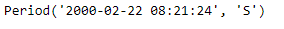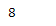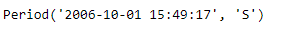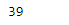# Python | Pandas Period.week

• Last Updated : 06 Jan, 2019

Python is a great language for doing data analysis, primarily because of the fantastic ecosystem of data-centric python packages. Pandas is one of those packages and makes importing and analyzing data much easier.

Pandas` Period.week` attribute returns an integer value which represents the week number in which the given period lies.

Attention geek! Strengthen your foundations with the Python Programming Foundation Course and learn the basics.

To begin with, your interview preparations Enhance your Data Structures concepts with the Python DS Course. And to begin with your Machine Learning Journey, join the Machine Learning - Basic Level Course

Syntax : Period.week

Parameters : None

Return : week of the year

Example #1: Use `Period.week` attribute to find out the week in which the given period object would lie.

 `# importing pandas as pd``import` `pandas as pd`` ` `# Create the Period object``prd ``=` `pd.Period(freq ``=``'S'``, year ``=` `2000``, month ``=` `2``, day ``=` `22``, ``                        ``hour ``=` `8``, minute ``=` `21``, second ``=` `24``)`` ` `# Print the Period object``print``(prd)`

Output :Now we will use the `Period.week` attribute to find the week in which prd object lies.

 `# return the week value``prd.week`

Output :As we can see in the output, the `Period.week `attribute has returned 8 indicating the following period object lies in the 8th week of the given year.

Example #2: Use `Period.week` attribute to find out the week in which the given period object would lie.

 `# importing pandas as pd``import` `pandas as pd`` ` `# Create the Period object``prd ``=` `pd.Period(freq ``=``'S'``, year ``=` `2006``, month ``=` `10``,``               ``hour ``=` `15``, minute ``=` `49``, second ``=` `17``)`` ` `# Print the object``print``(prd)`

Output :Now we will use the `Period.week` attribute to find the week in which prd object lies.

 `# return the week value``prd.week`

Output :As we can see in the output, the `Period.week `attribute has returned 39 indicating the following period object lies in the 39th week of the given year.

My Personal Notes arrow_drop_up